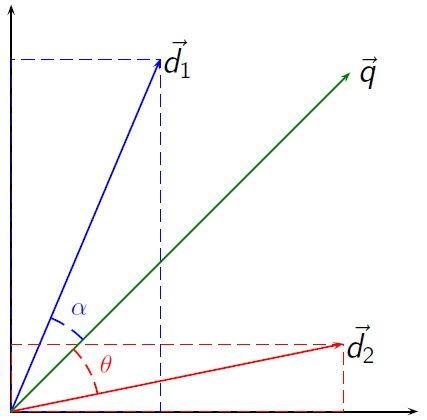# Vector Space Model: Examples

Instructor: Paul Bohan-Broderick

Paul has been teaching many subjects in many different ways since he received his PhD in 2001.

Vector space models are representations built from vectors. These (relatively) simple models are especially good at representing phenomena that are not usually considered numerical and have been utilized in unexpected domains such as literary criticism.

## Beyond the Numbers

Computers are tools for doing arithmetic-- adding, subtracting, multiplying and dividing integers. How can they also be powerful tools for learning about things that are not numerical?One powerful answer is a vector space model. A vector is a number that has both a magnitude and a direction. Both magnitude and direction need to be measured with respect to the space in which the vector is defined. Each dimension of the space represents a feature of interest and a vector represents the extent to which the object of the model has those features. Thus, a vector is a list of numbers: one for each feature that is part of the model space. The direction of the vector is the one that from the origin of the space to the point defined by those numbers.

While at first this might sound very difficult and complex, you will see in the following sections that the vector space model can be applied to a wide variety of different contexts.

## Physics

The easiest vector models to visualize come from physics. The most obvious vector model might describe the position of a particle in physical space using three numbers corresponding to measurements on three axes (length, depth and height). A more complicated physical vector model might include the three spatial dimensions and three dimensions corresponding to the speed in each of those directions. For example, a car might be one mile north and two mile east of my current location, but at the same elevation. The position of this car could be represented with the vector <1,2,0>. The first number represents position on the North-South axis, the second the position on the East-West access and the third represents positions on the up-down axis. If that car was travelling 60 mph due north, we could represent that as vector <1,2,0,60,0,0>, where the last three numbers represent speed in each of the three directions. In this example, if we had vectors representing different cars, we could compute their relative positions and velocities using trigonometry.

## Simple Texts

A text document can be represented as a vector. The vector space is defined by the terms that may be present in the text. For instance, each dimension in the space may represent a term in a dictionary of English. The dictionary defines the space. A text written in English would be a vector with a certain magnitude in each direction equal to the number of times that word or term appears.

If the word 'fish' appeared three times in a sentence, the vector would have a magnitude 3 in the fish direction. In a model space with the dimensions or features 'one', 'two', 'three', 'red', 'green', 'blue', and 'fish', the title of the Dr. Seuss classic One Fish, Two Fish, Red Fish, Blue Fish could be represented as <1,1,0,1,0,1,3>.

Many of Dr. Seuss' books are purposefully written with a vocabulary of only 50 words. Each of these books could be represented as a vector in 50-dimensional space or as a list of 50 numbers. Shakespeare's writing on the other hand contains around 28,829 specific words (or word types) and the vectors representing each play would be a very long lists of numbers.

Obviously, it is more rewarding to read a text rather than scan a list of numbers. However, representing a text in this way has many powerful applications, we will now look at two.

## Comparing Texts

Representing texts as vectors allows an interested user to compute the similarity of two texts in the same way that the relative positions and velocities of two cars could be computed in the first example. Vector representations of texts make it relatively easy to measure the similarity of different texts by comparing the angles between the vectors that represent each text.

A program might find that One Fish, Two Fish, Red Fish, Blue Fish has some important similarities to One, Two Buckle My Shoe because they have similar, or close, vector representations.

To unlock this lesson you must be a Study.com Member.

### Register to view this lesson

Are you a student or a teacher?

### Unlock Your Education

#### See for yourself why 30 million people use Study.com

##### Become a Study.com member and start learning now.
Back
What teachers are saying about Study.com

### Earning College Credit

Did you know… We have over 160 college courses that prepare you to earn credit by exam that is accepted by over 1,500 colleges and universities. You can test out of the first two years of college and save thousands off your degree. Anyone can earn credit-by-exam regardless of age or education level.